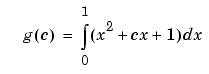ProgrammingExamples of Anonymous Functions

This section shows a few examples of how you can use anonymous functions. These examples are intended to show you how to program with this type of function. For more mathematically oriented examples, see the MATLAB Mathematics documentation.

The examples in this section include

Example 1 -- Passing a Function to quad

The equation shown here has one variable `t` that can vary each time you call the function, and two additional variables, `g` and `omega`. Leaving these two variables flexible allows you to avoid having to hardcode values for them in the function definition:

• ```x = g * cos(omega * t)
```

One way to program this equation is to write an M-file function, and then create a function handle for it so that you can pass the function to other functions, such as the MATLAB `quad` function as shown here. However, this requires creating and maintaining a new M-file for a purpose that is likely to be temporary, using a more complex calling syntax when calling `quad`, and passing the `g` and `omega` parameters on every call. Here is the function M-file:

• ```function f = vOut(t, g, omega)
f = g * cos(omega * t);
```

This code has to specify `g` and `omega` on each call:

• ```g = 2.5;  omega = 10;

quad(@vOut, 0, 7, [], [], g, omega)
ans =
0.1935

quad(@vOut, -5, 5, [], [], g, omega)
ans =
-0.1312
```

You can simplify this procedure by setting the values for `g` and `omega` just once at the start, constructing a function handle to an anonymous function that only lasts the duration of your MATLAB session, and using a simpler syntax when calling `quad`:

• ```g = 2.5;  omega = 10;

quad(@(t) (g * cos(omega * t)), 0, 7)
ans =
0.1935

quad(@(t) (g * cos(omega * t)), -5, 5)
ans =
-0.1312
```

To preserve an anonymous function from one MATLAB session to the next, `save` the function handle to a MAT-file

• ```save anon.mat f
```

and then `load` it into the MATLAB workspace in a later session:

• ```load anon.mat f
```

Example 2 -- Multiple Anonymous Functions

This example solves the following equation by combining two anonymous functions:

`    ``      ``      ``      ``      ``      `The equivalent anonymous function for this expression is

• ```g = @(c) (quad(@(x) (x.^2 + c*x + 1), 0, 1));
```

This was derived as follows. Take the parenthesized part of the equation (the integrand) and write it as an anonymous function. You don't need to assign the output to a variable as it will only be passed as input to the `quad` function:

• ```@(x) (x.^2 + c*x + 1)
```

Next, evaluate this function from zero to one by passing the function handle, shown here as the entire anonymous function, to `quad`:

• ```quad(@(x) (x.^2 + c*x + 1), 0, 1)
```

Supply the value for `c` by constructing an anonymous function for the entire equation and you are done:

• ```g = @(c) (quad(@(x) (x.^2 + c*x + 1), 0, 1));

g(2)
ans =
2.3333
```Variables Used in the Expression Primary M-File Functions© 1994-2005 The MathWorks, Inc.